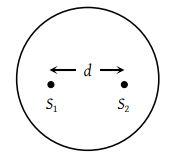Two coherent sources separated by distance d are radiating in phase having wavelength λ. A detector moves in a big circle around the two sources in the plane of the two sources. The angular position of n = 4 interference maxima is given as(1) ${\mathrm{sin}}^{-1}\frac{n\lambda }{d}$

(2) ${\mathrm{cos}}^{-1}\frac{4\lambda }{d}$

(3) ${\mathrm{tan}}^{-1}\frac{d}{4\lambda }$

(4) ${\mathrm{cos}}^{-1}\frac{\lambda }{4d}$

Explanation is a part of a Paid Course. To view Explanation Please buy the course.

Difficulty Level: